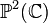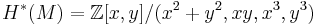# Connected sum of two complex projective planes with opposite orientation

(diff) ← Older revision | Latest revision (diff) | Newer revision → (diff)

## Definition

This topological space is defined as the connected sum of two copies of the complex projective plane$\mathbb{P}^2(\mathbb{C})$, where they are glued with opposite orientations.

## Algebraic topology

### Homology groups

The homology groups are as follows:$H_p(M) = \left\lbrace \begin{array}{rl}\mathbb{Z}, & \qquad p = 0,4 \\ \mathbb{Z} \oplus \mathbb{Z}, & \qquad p = 2 \\ 0, & \qquad \operatorname{otherwise}\\\end{array}\right.$

### Cohomology groups

The cohomology groups are as follows:$H^p(M) = \left\lbrace \begin{array}{rl}\mathbb{Z}, & \qquad p = 0,4 \\ \mathbb{Z} \oplus \mathbb{Z}, & \qquad p = 2 \\ 0, & \qquad \operatorname{otherwise}\\\end{array}\right.$

The cohomology ring is as follows:$H^*(M) = \mathbb{Z}[x,y]/(x^2 + y^2, xy, x^3,y^3)$

### Homotopy groups

The fundamental group is the trivial group.